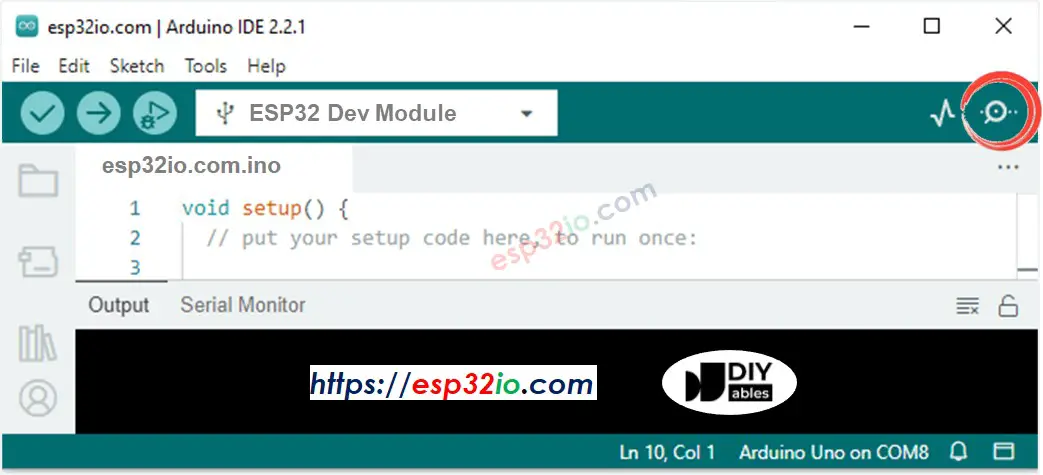# ESP32 - Potentiometer fade LED

This tutorial instructs you how to use ESP32 with the potentiometer to change the brightness of LED.

If you want to trigger LED when the voltage of potentiometer reaches a threshold, see ESP32 - potentiometer triggers LED tutorial

## Hardware Used In This Tutorial

Or you can buy the following sensor kit:

Disclosure: some of these links are affiliate links. We may earn a commission on your purchase at no extra cost to you. We appreciate it.

## Introduction to LED and Potentiometer

We have specific tutorials about LED and potentiometer. Each tutorial contains detailed information and step-by-step instructions about hardware pinout, working principle, wiring connection to ESP32, ESP32 code... Learn more about them at the following links:

## Wiring DiagramThis image is created using Fritzing. Click to enlarge image

If you're unfamiliar with how to supply power to the ESP32 and other components, you can find guidance in the following tutorial: How to Power ESP32.

## How To Program

• Reads the input on analog pin (value between 0 and 4095)
• Scales it to brightness (value between 0 and 255)
int brightness = map(analogValue, 0, 4095, 0, 255);
• Sets the brightness LED
analogWrite(LED_PIN, brightness);

## ESP32 Code

/* * This ESP32 code is created by esp32io.com * * This ESP32 code is released in the public domain * * For more detail (instruction and wiring diagram), visit https://esp32io.com/tutorials/esp32-potentiometer-fade-led */ #define POTENTIOMETER_PIN 36 // ESP32 pin GPIO36 (ADC0) connected to Potentiometer pin #define LED_PIN 21 // ESP32 pin GPIO21 connected to LED's pin // the setup routine runs once when you press reset: void setup() { // initialize serial communication at 9600 bits per second: Serial.begin(9600); // declare LED pin to be an output: pinMode(LED_PIN, OUTPUT); } // the loop routine runs over and over again forever: void loop() { // reads the input on analog pin A0 (value between 0 and 4095) int analogValue = analogRead(POTENTIOMETER_PIN); // scales it to brightness (value between 0 and 255) int brightness = map(analogValue, 0, 4095, 0, 255); // sets the brightness LED that connects to pin 3 analogWrite(LED_PIN, brightness); // print out the value Serial.print("Analog value = "); Serial.print(analogValue); Serial.print(" => brightness = "); Serial.println(brightness); delay(100); }

### Quick Instructions

• If this is the first time you use ESP32, see how to setup environment for ESP32 on Arduino IDE.
• Copy the above code and paste it to Arduino IDE.
• Compile and upload code to ESP32 board by clicking Upload button on Arduino IDE
• Open Serial Monitor on Arduino IDE• Rotate the potentiometer
• See the result on Serial Monitor. It looks like the below:
COM6
Analog value = 6 => brightness = 1 Analog value = 34 => brightness = 8 Analog value = 89 => brightness = 22 Analog value = 149 => brightness = 37 Analog value = 214 => brightness = 53 Analog value = 297 => brightness = 74 Analog value = 365 => brightness = 90 Analog value = 431 => brightness = 107 Analog value = 510 => brightness = 127 Analog value = 589 => brightness = 146 Analog value = 695 => brightness = 173 Analog value = 790 => brightness = 196 Analog value = 970 => brightness = 241 Analog value = 996 => brightness = 248 Analog value = 1018 => brightness = 253 Analog value = 4095 => brightness = 255
Autoscroll Show timestamp
Clear output
9600 baud
Newline

## Video Tutorial

Making video is a time-consuming work. If the video tutorial is necessary for your learning, please let us know by subscribing to our YouTube channel , If the demand for video is high, we will make the video tutorial.

※ OUR MESSAGES